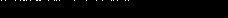not saved

# Hamming fury

We were a bit disappointed when we solved it 15 minutes after the end of the CTF (modulo RER transport), but however happy to find the flag.

## Resolutionfor this challenge we usually go through stegsolve in order to check the aspect of last bit planes. Unfortunately, it didn’t give anything. So then we tried to find the original image, and we found an image which had exactly the same dimensions.Then we xored original with steganoed one. And, oh wait, surprise ! Only a little part (written catchphrase excepted) of the first line was changed (and only LSB).So we can deduce that the message is here, in the first LSB of the image.

Then we remember that the description of the challenge was talking about “Hamming” and “F5 algorithm”.  And it was a chance, because I recently did a little work about this kind of steganography, which can be found at https://github.com/Alkanoor/Hamming_stegano.

The principle of this method is to use the power of coding theory, which allows us to create a message with very few modifications of the initial support.

This is (very briefly explained) how it works, with Hamming encoding :

Take a message m = [0 1 0]
Take a support x = [0 0 1 1 0 1 0]
Take a valid control matrix H of the code (you can check on the internet what that is, because I would explain it so badly you wouldn’t understand anything) : for example with Hamming code with parameters (7,4,3) :
H = [1 1 0 1 1 0 0]
[1 0 1 1 0 1 0]
[0 1 1 1 0 0 1]

Then compute m-Hx. Here it gives [1 0 0], which corresponds to the 5th column of H. So in our steganoed support we’ll change the 5th bit : x becomes y = [0 0 1 1 1 1 0].

So, we modified only one bit out of 7, and we convey a 3 bits message ! Check if we can easily find the hidden message with y (which is our new image) :
Hy = [0 1 0] = m
We did it !

So the same principle is used here. But obviously, we don’t know neither the parameters of the code (which define the size of H), nor H, nor bit plans order. We will have to bruteforce them, even if it isn’t very long.

Here is the python code which was used in order to find the flag. The image which is used here is only made of the first pixels of the left corner of steganoed image. Because of lack of time, we only tested with 2×3 hamming matrix instead of testing 3×7 in order to find the solution. In this final code, only good sized matrix are tested (and in a dirty way).

```from PIL import Image

im = Image.open('cool.png')

s = im.size
pix = [[() for i in range(s)] for j in range(s)]
for i in range(s):
for j in range(s):
pix[i][j] = im.getpixel((j,i))

h = len(rgb)
w = len(rgb)
res = *h
for i in range(h):
res[i] = *w
for j in range(w):
res[i][j] = []
for i in range(h):
for j in range(w):
for k in range(3):
for l in range(8):
if rgb[i][j][k]&(1<<l) > 0:
res[i][j].append(1)
else:
res[i][j].append(0)
return res

def findInMotif(mat_motif,order_target):
map = {}
for i in range(len(mat_motif)):
for j in range(len(mat_motif[i])):
map[mat_motif[i][j]] = (i,j)
return [map[order_target[i]] for i in range(len(order_target))]

def aggregate(data,mat_motif,order_target):
points = findInMotif(mat_motif,order_target)
res = []
n = len(data)
h = len(mat_motif)
w = len(mat_motif)//n
for i in range(0,len(data),h):
for j in range(0,len(data[i]),w):
for k in range(len(points)):
res.append(data[i+points[k]][j+points[k]//n][points[k]%n])
return res

d = convertToBitset(pix,[1,0,0,0,0,0,0,0,1,0,0,0,0,0,0,0,1,0,0,0,0,0,0,0])

import matplotlib.pyplot as plt
import numpy as np
import binascii

def binarize_array(a):
for i in range(len(a)):
a[i] = a[i]%2
return a

import itertools

def recov(lsb):
n = 7
k = 3
possib = [[[1,0,1],[1,1,0]],[[1,0,1],[0,1,1]],[[1,1,0],[1,0,1]],[[1,1,0],[0,1,1]],[[0,1,1],[1,1,0]],[[0,1,1],[1,0,1]]]
possib = [[1,1,0],[1,0,1],[0,1,1],[1,1,1],[1,0,0],[0,1,0],[0,0,1]]

perm = itertools.permutations(range(7))
for L in perm:
H = np.array([possib[i] for i in L]).T
cur_byte = ''
ret = ''

for i in range(0,len(lsb),n):
y = lsb[i:i+n]

for j in range(len(y),n):
y = np.append(y,0)

m = binarize_array(H.dot(y))

for j in m:
cur_byte += chr(j+ord('0'))
while len(cur_byte)>8:
ret += chr(int(cur_byte[:8],2))
if ret[len(ret)-1] == '\x00':
pass
#print(ret)
cur_byte = cur_byte[8:]
if ret[:2]=='nd':
print(ret)
print(H)
print(L)

possibilities = [[0,1,2],[0,2,1],[1,0,2],[1,2,0],[2,0,1],[2,1,0]]
#possibilities = [[0,1,2,3,4,5],[0,2,1,3,5,4],[1,0,2,4,3,5],[1,2,0,4,5,3],[2,0,1,5,3,4],[2,1,0,5,4,3],[0,1,3,4],[0,2,3,5],[1,2,4,5],[1,0,4,3],[2,0,5,3],[2,1,5,4]]

for p in possibilities:
c = aggregate(d,[[0,1,2]],p)
recov(c)
```

Here is the zip which contains everything that is useful (except original images which are too heavy) : hammingFury

We got the flag : ndh2k16_ea5tc057dada2F.